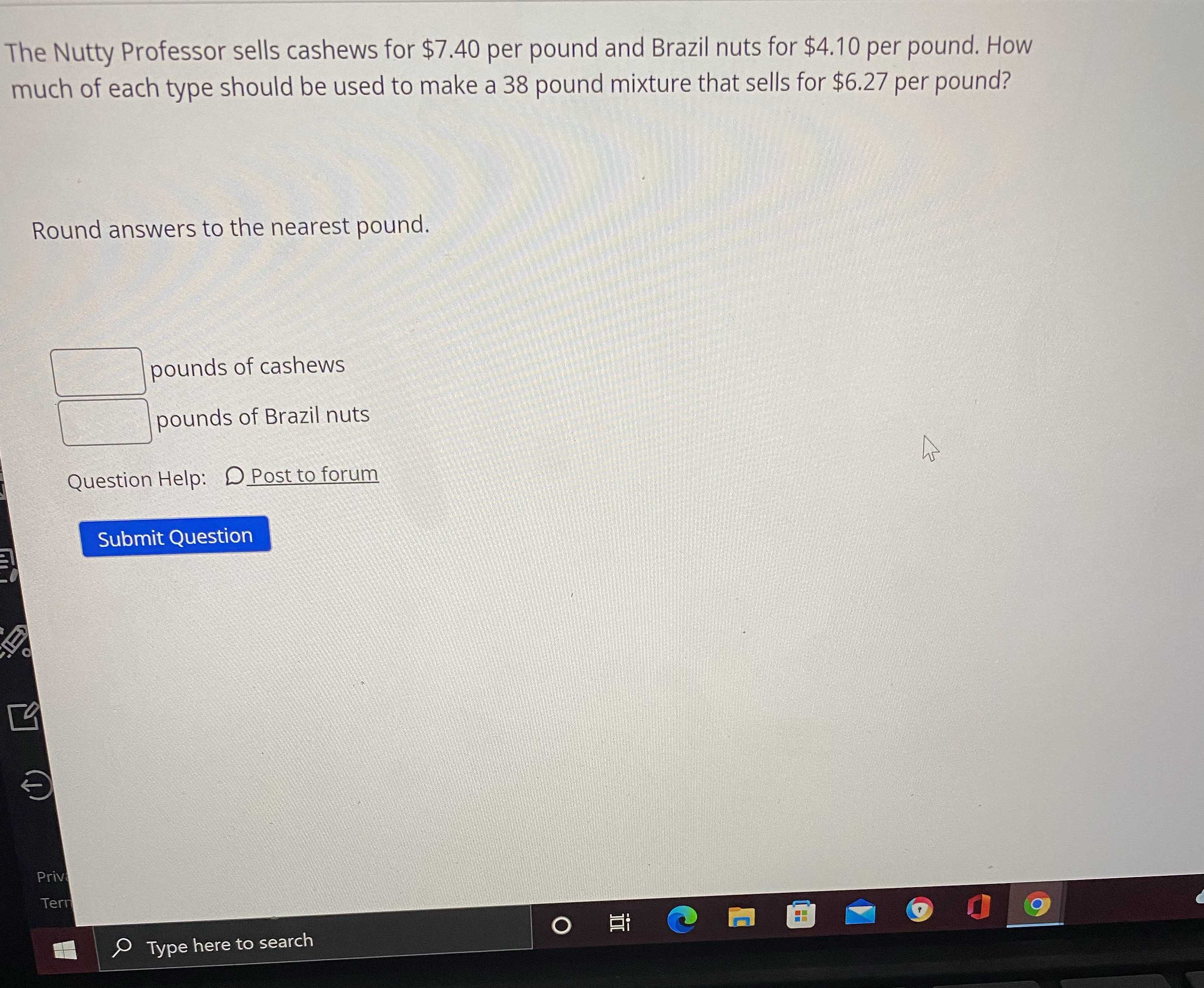### Still have math questions?

Algebra
QuestionThe Nutty Professor sells cashews for $$\ 7.40$$ per pound and Brazil nuts for $$\ 4.10$$ per pound. How much of each type should be used to make a $$38$$ pound mixture that sells for $$\ 6.27$$ per pound?

Round answers to the nearest pound.

_____pounds of cashews

_____pounds of Brazil nuts